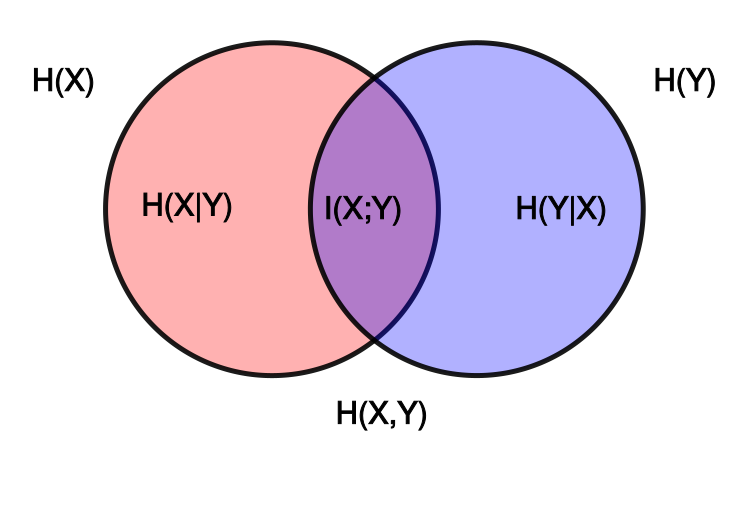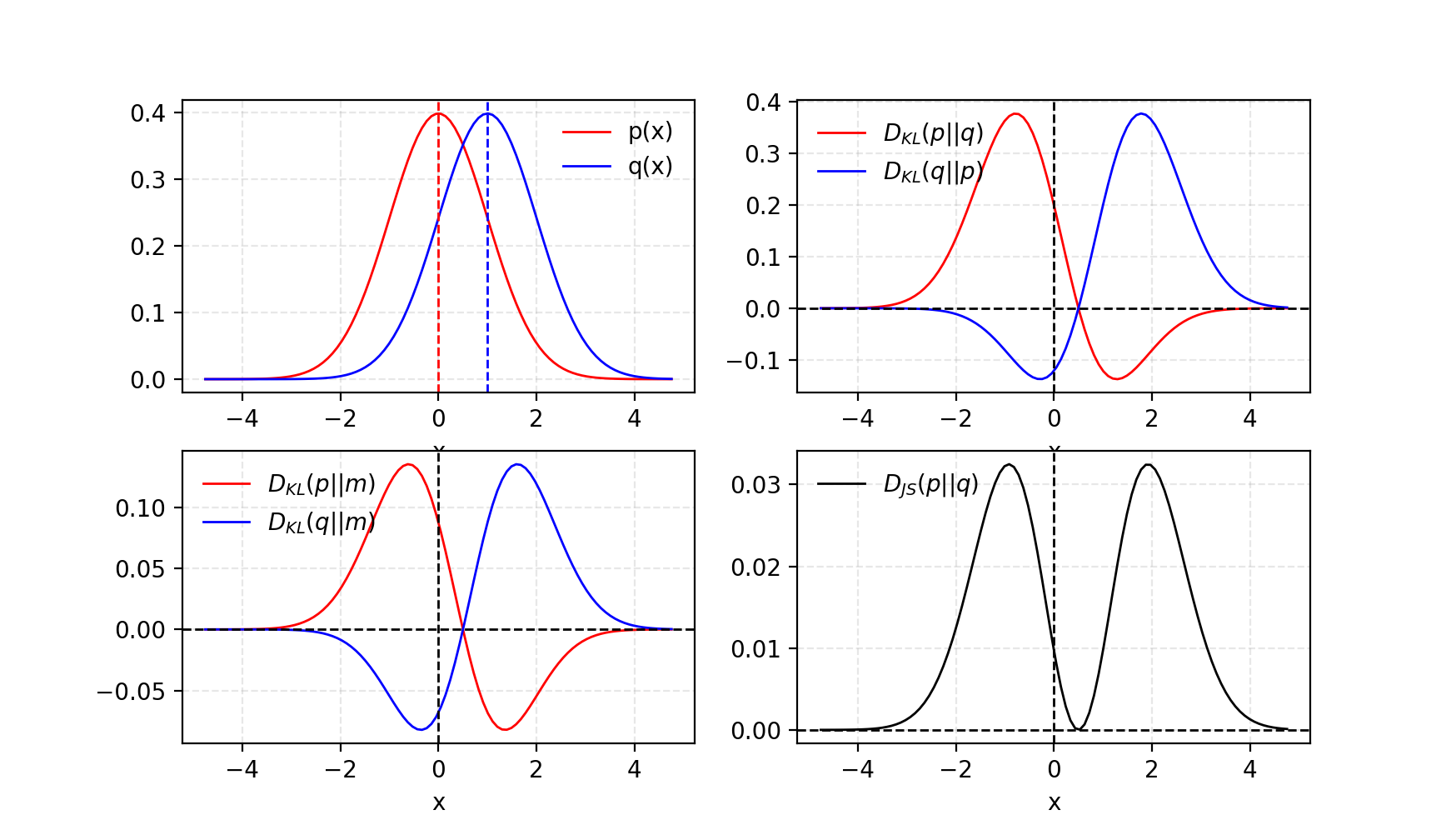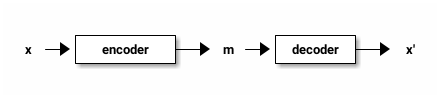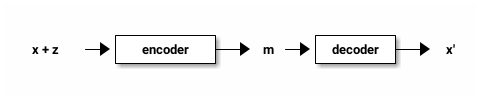# Understand Deep Generative Models from Ground Up

## Preface

The lecture has 40 min time limit and we have length slides to consume. We may skip some pages if we have to :3.

## Mathematical Foundations

In this section, we will have brief intro on information theory. Then we dive into these concepts that are fundamental to deep generative models.

• KL-divergence

• Jensen–Shannon divergence

## Information Theory - Recap

• The amount of information can be understood as the surpriseness of an event.

• That is, rare events give us more information. We need more bits to encode that event in terms of binary encoding.

• Suppose we have a fair dice, which is represented by a rv $X$ with events $\{1, 2, 3, 4, 5, 6\}$. The amount of information of event $X = 3$ is measured by $I(X = 3) = \log_2 \frac{1}{P(X = 3)} \approx 2.58$.

## Information Theory - Information Content

• The amount of information can be intuitively understood as # of perfect queries to locate that event.

• Suppose our $X = 3$ example. We ask three yes-no queries to find it out.

• Is it greater than or equal to 4? No

• Is it greater or equal to 2? Yes

• Is it 3? Yes

• With perfect yes-no query, it is expected to ask $\log_2 \frac{1}{P(X = 3)}$ questions.

## Information Theory - Information Content

• In fact, we need law of large numbers and many theories to show this idea. It is out of scope in this lecture.

• The information content is additive on individual events. Suppose we roll the dice twice. The information content of joint event $X_1 = 3, X_2 = 2$ is

\begin{aligned} \log_2 \frac{1}{P(X_1 = 3, X_2 = 2)} \\ = \log_2 \frac{1}{P(X_1 = 3)} + \log_2 \frac{1}{P(X_2 = 2)} \\ = I(X_1 = 3) + I(X_2 = 2) \end{aligned}

## Information Theory - Entropy

• The (Shannon) entropy of rv $X$, denoted as $H(x)$, can be understood as expected amount of information.

• Suppose a rv $X$ and its pdf/pmf $P(x)$.

\begin{aligned} H(X) \ \\ = E_{X \sim P}[I(X)] \ \\ = \sum_x P(x) \log_b \frac{1}{P(x)} \ \text{(discrete)} \\ = \int_x P(x) \log_b \frac{1}{P(x)} \ \text{(continuous)} \end{aligned}
• The entropy is usually interpreted as the randomness of a random variable.

## Information Theory - Information Gain

• What if we encode a true distribution $P$ with artifact distribution $Q$?

• Encoding based on $Q$ can be thought of as an imperfect query for $P$.

• The difference $E_{x \sim P(x)}[\frac{1}{Q(x)}] - E_{x \sim P(x)}[\frac{1}{P(x)}]$ is always non-negative due to Gibb’s inequality.

## Information Theory - Conditional Entropy

• Suppose you have the knowledge of rv $Y$, and would like to know the randomness of rv $X$. It can be modeled as conditional entropy $H(X|Y)$.

• The rationale goes as following:

• Given value $y$, $H(X | Y = y) = \sum_x P(X = x | Y = y) \log \frac{1}{P(X = x | Y = y)}$

• $H(X|Y) = \sum_y P(y) H (X | Y = y)$ is expected value over $y$.

## Information Theory - Conditional Entropy

• $H(X | Y)$ is smaller than or equal to $H(X)$ because you have prior knowledge of $Y$.

• For example, $X$ indicates whether teacher is inside R442, and $Y$ is whether you observe teacher walk into R442.

• $H(x) \gt 0$ because you cannot make sure his or her existence. $H(X|Y)$ is strictly less than $H(X)$ because you know the outcome of $X$ if $Y = \text{true}$.

• $H(X) = H(X|Y)$ if $X$ and $Y$ are individual events.

## Information Theory - Mutual Information

• The dependence of rvs $X, Y$ is modeled as mutual information $I(X; Y)$.

• In fact,

\begin{aligned} I(X; Y) \\ = H(X) - H(X | Y) \\ = H(Y) - H(Y | X) \\ = H(X) + H(Y) - H(X, Y) \\ = H(X, Y) - H(X|Y) - H(Y|X) \end{aligned}

## Information Theory - Mutual Information## Kullback–Leibler Divergence

• Suppose two probability distributions $P$ and $Q$. The Kullback–Leibler divergence of $P$ with respect to $Q$ is formulated as:

$D_{\text{KL}} (P \parallel Q) = E_{x \sim P}[\log \frac{1}{Q(x)}] - E_{x \sim P}[\log \frac{1}{P(x)}]$

## Kullback–Leibler Divergence - Interpretations

• $P$ is regarded as true probability, and $Q$ is regarded as theory.

• In coding theory, it measures expected # of extra bits to encode samples from $P$ using code optimized for $Q$.

• In Bayesian inference, $Q$ is prior and $P$ is posterior. In detail,

• $Q = p(\theta)$ the probability over our model parameters $\theta$.

• $P = p(\theta | x)$ give a new observation $x$.

• The divergence is information gain by revising the belief $Q$ to $P$.

## Kullback–Leibler Divergence & Jensen–Shannon Divergence

• KL-div is not symmetric! $D_{\text{KL}(P \| Q)}$ may not be the same with $D_{\text{KL}(Q \| P)}$.

• Jensen–Shannon divergence fixes this by

$D_{\text{JS}} (P \| Q) = \frac{1}{2} D_{\text{JS}} (P \| M) + \frac{1}{2} D_{\text{JS}} (Q \| M)$

where $M = \frac{1}{2}(P + Q)$

## Kullback–Leibler Divergence & Jensen–Shannon Divergence## Remarks

• KL-divergence should never be misnamed as KL-metric or KL-distance. Since it is not symmetric, it does not fit the mathematical definition of metric.

• $D_{\text{KL}}(P \| Q)$ could be infinite if $P(x) \gt 0$ and $Q(x) = 0$ for some $x$.

• Both KL-div and JS-div does not respect the metric on support, leading to vanishing gradient.

• We will introduce Watterstein metric to resolve these issues. === Conclusion

• Understand basics of information theory and intuition behind KL-divergence.

• Knows Jensen-Shannon divergence.

## Variational Autoencoder (VAE)

• Vanilla autoencoder and noise autoencoder

• Gaussian mixture model

• Evidence lower bound (ELBO)

## Autoencoder

• Intuition: Given data sample $x$, encode it into latent space code $m$. Then decode it into reconstruction $x'$.

• Trained by minimizing the difference b/w input and reconstruction $L(x, x')$, usually by a L2 or cross-entropy.

• We can represent $x$ by lower dimensional code $m$.## Noisy Autoencoder

• The autoencoder may not be robust to slight changes on input $x$.

• One solution is to add some noise $z$ on input $x$.## Deriving VAE

• Can we slightly change the code $m$ to $m'$, and generate new reasonable sample by decoding $m'$?

• In fact, it does not work as expected, because both encoder and decoder are non-linear. We cannot expect the latent space has that good property.

• Solution: add some noise $z$ on latent code $m$.